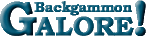Cubeless EquityCubeless Equity The value of a position if the game is played without a doubling cube. This is a value between -3 and +3 and is equal to P(W) + P(Wg) + P(Wbg) - P(L) - P(Lg) - P(Lbg), where P(W) is the probability of winning the game, P(Wg) is the probability of winning a gammon (or backgammon), P(Wbg) is the probability of winning a backgammon, P(L) is the probability of losing the game, P(Lg) is the probability of losing a gammon (or backgammon), P(Lbg) is the probability of losing a backgammon.  Compare: Cubeful Equity.

 Help   •   About the Glossary   •   List of Terms   •   Full Glossary